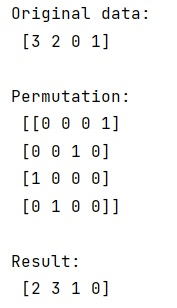# How to invert a permutation array in NumPy?

Learn, how can we invert a permutation array in Python NumPy?
Submitted by Pranit Sharma, on March 20, 2023

## Inverting a permutation NumPy Array

Suppose that we are given a numpy array and we perform some kind of permutation on it, and we need to create an array to represent the inverse of this permutation.

To invert a permutation array in NumPy, we can use numpy.where(p.T) where p is the permutation on the array and T is the transpose of the new array.

Let us understand with the help of an example,

## Python code to invert a permutation array in NumPy

```# Import numpy
import numpy as np

# Creating a numpy array
arr = np.array([3,2,0,1])
row = np.arange(4)

# Display original data
print("Original data:\n",arr,"\n")

# Permutation
p = np.zeros((4,4),dtype=int)
p[row,arr] = 1

# Inverse of permutation
res = np.where(p.T)

# Display permutation
print("Permutation:\n",p,"\n")

# Display result
print("Result:\n",res,"\n")
```

### Output